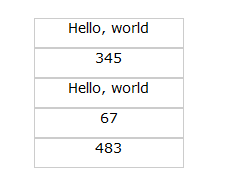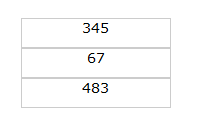# JavaScript函数式编程

## JavaScript 中的函数式编程

JavaScript 是一门被误解甚深的语言，由于早期的 Web 开发中，充满了大量的 copy-paste 代码，因此平时可以见到的 JavaScript 代码质量多半不高，而且 JavaScript 代码总是很飞动的不断闪烁的 gif 广告，限制网页内容的复制等联系在一起的，因此包括 Web 开发者在内的很多人根本不愿意去学习 JavaScript。 这种情形在 Ajax 复兴时得到了彻底的扭转，Google Map，Gmail 等 Ajax 应用的出现使人们惊叹：原来 JavaScript 还可以做这样的事！很快，大量优秀的 JavaScript/Ajax 框架不断出现，比如 Dojo，Prototype，jQuery，ExtJS 等等。这些代码在给页面带来绚丽的效果的同时，也让开发者看到函数式语言代码的优雅。

### 函数式编程风格

##### 清单 4. LISP 中的加法
` (+ 1 3 4 5 6 7)`

##### 清单 5. JavaScript 中的求和
```function sum(){
var res = 0;
for ( var i = 0, len = arguments.length; i < len; i++){
res += parseInt(arguments[i]);
}
return res;
}

print(sum(1,2,3));
print(sum(1,2,3,4,6,7,8));```

``` 6
31```

##### 清单 6. 一些简单的函数抽象
```  function add(a, b){  return a+b; }
function sub(a, b){  return a-b; }
function mul(a, b){  return a*b; }
function div(a, b){  return a/b; }
function rem(a, b){  return a%b; }
function inc(x){  return x + 1; }
function dec(x){  return x - 1; }
function equal(a, b){  return a==b; }
function great(a, b){  return a>b; }
function less(a, b){  return a<b; }```

##### 清单 7. 函数式编程风格
``` // 修改之前的代码
function factorial(n){
if (n == 1){
return 1;
} else {
return factorial(n - 1) * n;
}
}

// 更接近“函数式”编程风格的代码
function factorial(n){
if (equal(n, 1)){
return 1;
} else {
return mul(n, factorial(dec(n)));
}
}```

### 闭包及其使用

##### 清单 8. 一个闭包的例子
```function outter(){
var n = 0;
return
function (){
return n++;
}
}

var o1 = outter();
o1();//n == 0
o1();//n == 1
o1();//n == 2
var o2 = outter();
o2();//n == 0
o2();//n == 1```

##### 清单 9. jQuery 中的闭包
``` var con = \$("div#con");
setTimeout( function (){
con.css({background:"gray"});
}, 2000);```

##### 清单 10. 错误的使用闭包
```  var outter = [];
function clouseTest () {
var array = ["one", "two", "three", "four"];
for ( var i = 0; i < array.length;i++){
var x = {};
x.no = i;
x.text = array[i];
x.invoke =  function (){
print(i);
}
outter.push(x);
}
}```

##### 清单 11. 匿名对象
``` {
no : Number,
text : String,
invoke :  function (){
// 打印自己的 no 字段
}
}```

##### 清单 12. 错误的结果
``` clouseTest();// 调用这个函数，向 outter 数组中添加对象
for ( var i = 0, len = outter.length; i < len; i++){
outter[i].invoke();
}```

``` 4
4
4
4```

##### 清单 13. 错误的原因
```function invoke(){
print(i);
}```

##### 清单 14. 正确的使用闭包
``` var outter = [];
function clouseTest2(){
var array = ["one", "two", "three", "four"];
for ( var i = 0; i < array.length;i++){
var x = {};
x.no = i;
x.text = array[i];
x.invoke =  function (no){
return
function (){
print(no);
}
}(i);
outter.push(x);
}
}```

``` //x == 0
x.invoke =  function (){print(0);}
//x == 1
x.invoke =  function (){print(1);}
//x == 2
x.invoke =  function (){print(2);}
//x == 3
x.invoke =  function (){print(3);}```

## 实际应用中的例子

##### 清单 15. jQuery 选择器
```  var cons = \$("div.note");// 找出所有具有 note 类的 div
var con = \$("div#con");// 找出 id 为 con 的 div 元素

##### 清单 16. jQuery 操作 jQuery 对象 (List)
``` cons.each( function (index){
\$( this ).click( function (){
//do something with the node
});
});```

##### 清单 17. 扩大 / 缩小 jQuery 集合
``` cons.find("span.title");// 在 div.note 中进行更细的筛选
cons.add("div.warn");// 将 div.note 和 div.warn 合并起来
cons.slice(0, 5);// 获取 cons 的一个子集```

##### 清单 18. 页面的 HTML 结构
``` <div>
<span>Hello, world</span>
</div>
<div>
<span>345</span>
</div>
<div>
<span>Hello, world</span>
</div>
<div>
<span>67</span>
</div>
<div>
<span>483</span>
</div>```

##### 图 1. 过滤之前的效果我们通过 jQuery 对包装集进行一次过滤，jQuery 的过滤函数可以使得选择出来的列表对象只保留符合条件的，在这个例子中，我们保留这样的 div，当且仅当这个 div 中包含一个类名为 title 的 span，并且这个 span 的内容为数字：
##### 清单 19. 过滤集合
``` var cons = \$("div.note").hide();// 选择 note 类的 div, 并隐藏
cons.filter( function (){
return \$( this ).find("span.title").html().match(/^d+\$/);
}).show();```

##### 图 2. 过滤之后的效果我们再来看看 jQuery 中对数组的操作 ( 本质上来讲，JavaScript 中的数组跟 List 是很类似的 )，比如我们在前面的例子中提到的 map 函数，过滤器等：
##### 清单 20. jQuery 对数组的函数式操作
``` var mapped = \$.map([1, 2, 3, 4, 5, 6, 7, 8, 9, 10],
function (n){
return n + 1;
});
var greped = \$.grep([1, 2, 3, 4, 5, 6, 7, 8, 9, 10],
function (n){
return n % 2 == 0;
});```
mapped 将被赋值为 :
` [2, 3, 4, 5, 6, 7, 8, 9, 10, 11]`

` [2, 4, 6, 8, 10]`

##### 清单 21. 一个页面刷新的例子
```function update(item){
return
function (text){
\$("div#"+item).html(text);
}
}
function refresh(url, callback){
var params = {
type : "echo",
data : ""
};
\$.ajax({
type:"post",
url:url,
cache: false ,
async: true ,
dataType:"json",
data:params,

success:  function (data, status){
callback(data);
},

error:  function (err){